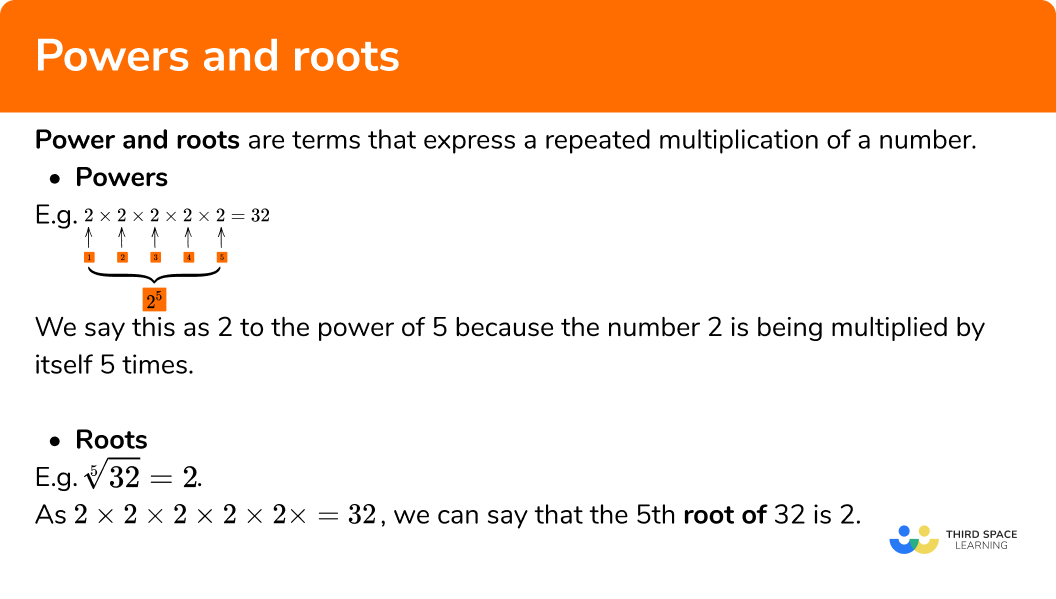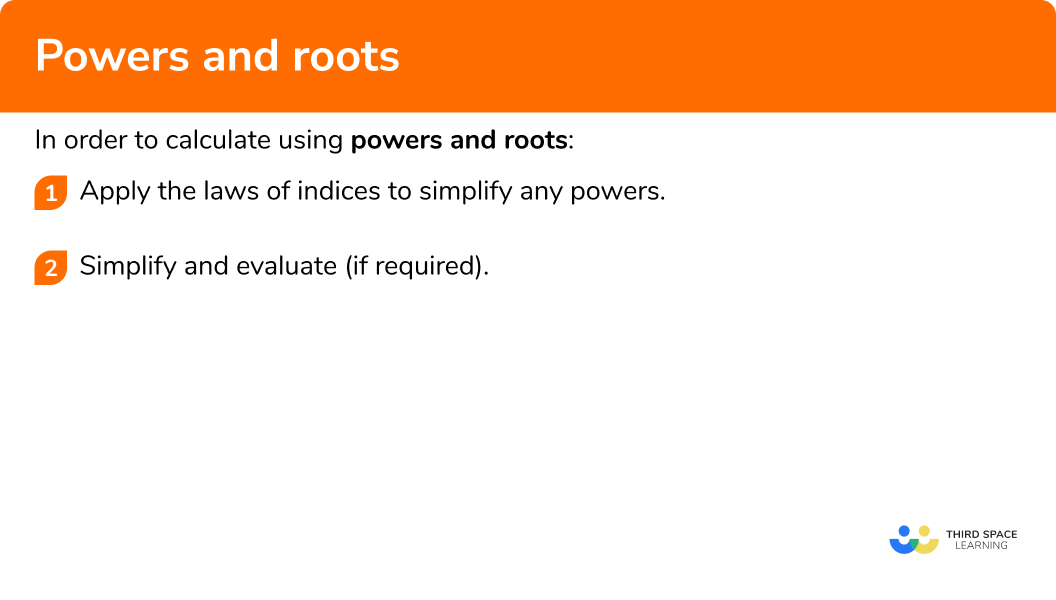GCSE Maths Number

Powers And Roots

# Powers And Roots

Here we will learn about powers and roots, including different types of powers, expressing a power with a different base and special cases.

There are also powers and roots worksheets based on Edexcel, AQA and OCR exam questions, along with further guidance on where to go next if you’re still stuck.

## What are powers and roots?

Power and roots are terms that express a repeated multiplication of a number.

• Powers

For example,

When calculating 2\times2\times2\times2\times2 , we can express the same calculation as 2^5 .

We say this as 2 to the power of 5 because the number 2 is being multiplied by itself 5 times.

• Roots

Let’s consider the inverse of this expression.

As 2\times2\times2\times2\times2=32 , we can say that the 5^\text{th} root of 32 is 2 .

This would be written as \sqrt{32}=2 .

Powers and roots separately are inverse operations (like addition and subtraction).

### What are powers and roots?## Powers of integers

Powers of integers involves raising an integer (whole number) to a power (also known as an exponent). When we have a positive power, the power tells us how many times we need to multiply the integer (also known as the base), by itself.

For example,

3^5

We have an integer base, 3, raised to a positive power, 5.

This means 3 \times 3 \times 3 \times 3 \times 3 = 243 .

When we have a negative power, it impacts the integer differently. When we raise an integer to a negative power, we need to find the positive reciprocal of the number.

For example,

3^{-3}

We have an integer base, 3, raised to a negative power, -3.

The reciprocal of 3 is \frac{1}{3} .

3^{-3} =\frac{1}{3^3} = \frac{1}{27}.

There are also some special cases to consider,

If we raise a non-negative number to the power of 0 the answer is always 1, regardless of what the base number is.

For example,

4^{0}= 1

9999^{0}= 1

Note: 0^0 is a very special case. There are still many disagreements about what its value should be, with some mathematicians saying 1 and others saying that it cannot be defined.

If we raise a number to the power of 1, it doesn’t do anything, nothing changes. Remember, the power tells us how many times we multiply a number by itself.

For example,

5^{1}= 5

199^{1}= 199

## Using powers and roots

We can use powers and roots in a variety of ways,

• Square numbers and square roots

A square number is a number that is multiplied by itself.

The square root of a number is a value that can be multiplied by itself to give the original number.

For example,

6^2=6\times6=36

\sqrt{121}=11

Step-by-step guide: Square numbers and square roots

• Cube numbers and cube roots

A cube number is found when we multiply an integer (whole number) by itself three times.

The cube root of a number is a value that can be multiplied by itself three times to give the original number.

For example,

7^3=343

\sqrt{64}=4

Step-by-step guide: Cube numbers and cube roots

• The powers of 0 and 1

For example,

6^0=1

4.8^1=4.8

Step-by-step guide: To the power of

• Negative powers

When a number is raised to a negative power, we calculate the positive reciprocal of that number.

For example,

10^{-2}=\frac{1}{10^2}=\frac{1}{100}

Step-by-step guide: Negative powers

• Fractional powers

Fractional powers are a type of index that represents the nth root of a number.

For example,

8^{\frac{1}{3}}=\sqrt{8}=2

Step-by-step guide: Fractional powers

• Expressing a power with a different base

We can sometimes express a power with a different base in order to help to simplify expressions.

For example,

8\times2^{2}=2^{3}\times2^{2}=2^5

Step-by-step guide: Expressing a power with a different base

• The laws of indices

Powers and roots are used in the five laws of indices,

Step-by-step guide: Laws of indices

## Keywords

There are lots of important words we need to know for powers and roots,

KeywordDefinition / Example
ExponentThe power p in an expression of the form a^{p}.

Related terms: power, exponential function, exponential, index.

For example,

3^{2}=3 \times 3 is the same as “3 to the power of 2” where 2 is the exponent.
BaseThe base a in an expression of the form a^{p}.

Related terms: whole number, decimal, integer.

For example,

5^{3}=5 \times 5 \times 5 is the same as “5 to the power of 3” where 5 is the base.
Index The index tells us the power or the number of times the base is multiplied by itself.

The plural of one index is multiple indices.

Related terms: power, exponent

For example,

4^{1}=4 where “1 in the index of 4”.
InverseThe inverse is used to describe an opposite function.

For example,

The inverse to addition is subtraction

The inverse to multiplication is division

The inverse to squaring a number is to square root a number.
BracketsBrackets are used to group numbers, operations or variables together.

Related terms: parentheses

For example,

2(x+3) is an expression where the number 2 is being multiplied by (x+3).
IntegerAn integer is a whole number.

For example,

4, 17, 203 are all integers.
SurdA surd is an unresolved root of the form \sqrt[n]{x}.

For example,

\sqrt{2} is the square root of 2, which is an irrational number.
Rational numberA rational number is a number that can be expressed as a fraction \frac{a}{b} where a, and b are integers, and b 0.

For example,

\frac{5}{8} is a rational number as 5 and 8 are integers, expressed as a fraction.
Irrational numberAn irrational number cannot be expressed as a fraction.

For example,

\pi is an irrational number as it cannot be expressed exactly as a fraction.

## How to calculate using powers and roots

In order to calculate using powers and roots:

1. Apply the laws of indices to simplify any powers.
2. Simplify and evaluate (if required).

### Explain how to calculate using powers and roots## Powers and roots examples

### Example 1: calculations including a fraction

Simplify and evaluate the expression,

\frac{5^{3}\times{5^{5}}}{5^{4}} .

1. Simplify any powers using laws of indices.

We can simplify the numerator of the fraction as x^{a}\times x^{b}=x^{a+b} , so we have

\frac{5^{3}\times{5^{5}}}{5^{4}}=\frac{5^{3+5}}{5^{4}}=\frac{5^{8}}{5^{4}}.

As the numerator and denominator of a fraction are divided, we can use

x^{a}\times x^{b}=x^{a-b} to obtain

\frac{5^{8}}{5^{4}}=5^{8-4}=5^{4}.

2Evaluate or solve (if required).

Evaluating the expression 5^{4} , we get

5^{4}=5\times5\times5\times5=625 .

So \frac{5^{3}\times{5^{5}}}{5^{4}}=625.

### Example 2: fractional indices

Evaluate the following expression,

(3^{4})^{\frac{1}{2}}\times{3} .

1. Simplify any powers using laws of indices.

We can simplify the term (3^{4})^{\frac{1}{2}} as (x^{a})^{b}=x^{ab} so we have,

(3^{4})^{\frac{1}{2}}=3^{4\times\frac{1}{2}}=3^{2} .

So we can now write (3^{4})^{\frac{1}{2}}\times{3}=3^{2}\times{3} .

2Evaluate or solve (if required).

As 3=3^{1} , we can evaluate the expression 3^{2}\times3 to get

3^{2}\times3=3^{2+1}=3^{3}=27.

So (3^{4})^{\frac{1}{2}}\times{3}=27.

### Example 3: roots

Simplify the following expression to a single power of 2 ,

\sqrt{4^{3}}\times\sqrt{8} .

1. Simplify any powers using laws of indices.

The first term in the expression is the square root of 4 cubed. This is equivalent to the square root of 4 , cubed as the powers are interchangeable,

\sqrt{4^{3}}=(\sqrt{4})^{3} .

This means that \sqrt{4^{3}}=(\sqrt{4})^{3}=2^{3}.

The next term is the cube root of 8 . As 8 is a cube number, we can state that \sqrt{8}=2 as 2^{3}=8.

So we can now write \sqrt{4^{3}}\times\sqrt{8}=2^{3}\times{2}.

2Evaluate or solve (if required).

2^{3}\times 2=2^{3+1}=2^{4}.

So \sqrt{4^{3}}\times\sqrt{8}=2^{4}.

### Example 4: algebraic

Simplify the expression,

f(x)=5x^{-2}\times 4x^{5} -2x .

1. Simplify any powers using laws of indices.

We can use the index law x^{a}\times x^{b}=x^{a+b} to partially simplify 5x^{-2}\times 4x^{5}-2x .

This is because we can only simplify the base numbers that are multiplied, 5x^{-2}\times 4x^{5}.

5x^{-2}\times 4x^{5} = 5\times 4\times x^{-2}\times x^{5} = 20\times x^{3}=20x^{3}.

So we now have 20x^{3}-2x .

2Evaluate or solve (if required).

If we knew a value for x , or f(x) we would continue to solve the equation however, for this question, we only need to state the simplified answer,

20x^{3}-2x.

### Example 5: fractional base

(\frac{c^{10}}{c^{4}})^{-\frac{1}{4}}

1. Simplify any powers using laws of indices.

As the numerator and denominator of the fraction are in terms of c , we can simplify this using the rule x^{a}\div x^{b}=x^{a-b} ,

c^{10}\div c^{4}=c^{10-4}=c^{6}.

We can then use the law of indices to remove the brackets (x^{a})^{b}=x^{ab},

(c^{6})^{-\frac{1}{4}}=c^{6\times{-\frac{1}{4}}}=c^{-\frac{6}{4}} .

2Evaluate or solve (if required).

As this expression is in terms of c , we can only complete the simplification of the expression by simplifying the fractional power,

c^{-\frac{6}{4}}=c^{-\frac{3}{2}} .

### Example 6: change the base

Write 2 as a power of 8 .

1. Simplify any powers using laws of indices.

As we want to express 2 as a power of 8 , we need to calculate how we can use powers to get from 8 to 2 .

We know that 2^{3}=8 and so \sqrt{8}=2.

2Evaluate or solve (if required).

Using the fact that x^{\frac{m}{n}}=\sqrt[n]{x^m} ,

we can say \sqrt{8}=8^{\frac{1}{3}}.

So 2=8^{\frac{1}{3}}.

### Common misconceptions

• BIDMAS

When raising a base to a power, the power is associated with a variable or an expression.

For example, let’s look at 3x^{-2}.

Using BIDMAS, we can see that x is being raised to the power of -2 , and then is multiplied by 3 to get the answer \frac{3}{x^{2}}.

However a common error is to calculate 3x raised to the power of -2 , giving the answer \frac{1}{9x^{2}} which is incorrect. If this was the case, the question would be written as (3x)^{-2} .

• Incorrect application of the exponent

A similar circumstance is applied to fractions that are raised to a power.

For example, let’s look at (\frac{5}{2})^{-3}.

The correct application of the laws of indices would give a correct answer of  (\frac{5}{2})^{-3}=(\frac{2}{5})^{3}=\frac{8}{125}.

However a common error would be to apply the -3 only to the numerator, leaving the denominator unchanged. This would give the incorrect answer of (\frac{5}{2})^{-3}=\frac{1}{125}\times\frac{1}{2}=\frac{1}{250}.

### Practice powers and roots questions

1. Express the following as a single power of 4 \frac{4^{8}\times{4^{4}}}{4^{-3}} .

4^{9}4^{15}4^{-4}4^{29}\frac{4^{8}\times{4^{4}}}{4^{-3}}=4^{8+4-{-3}}=4^{8+7}=4^{15}

2. Evaluate \frac{(5^{6})^{\frac{1}{3}}}{5} .

2125525\frac{(5^{6})^{\frac{1}{3}}}{5}=\frac{5^{6\times\frac{1}{3}}}{5}=\frac{5^{2}}{5}=5^{2-1}=5^{1}=5

3. Evaluate \sqrt{81}\div 9^{\frac{1}{2}} .

34.591\sqrt{81}\div 9^{\frac{1}{2}}=3\div{3}=1

4. Simplify f(y)=y+8y^{6}\div 2y^{3} .

4y^{3}+y4y^{4}5y^{3}4.5y^{2}f(y)=y+8y^{6}\div 2y^{3}=y+4y^{6-3}=y+4y^{3}

5. Simplify (\frac{x}{x^{-2}\times{x^{3}}})^{-4} .

-110x(\frac{x}{x^{-2}\times{x^{3}}})^{-4}=(x^{1-(-2+3)})^{-4}=(x^{0})^{-4}=x^{0}=1

3. Write 3 as a power of 27 .

3^{3}27^{-3}27^{\frac{1}{3}}3### Powers and roots GCSE questions

1. Simplify the expression 4x^{2}\times 3x^{5} .

(1 mark)

12x^{7}

(1)

2. (a) Simplify f(x)=(\frac{1}{9x^{2}})^{-2} .

(b) Hence or otherwise, evaluate f(3^{-1}) .

(6 marks)

(a)

\frac{1}{9x^{2}}=(9x^{2})^{-1}

(1)

((9x^{2})^{-1})^{-2}=(9x^{2})^{-1\times{-2}}=(9x^{2})^{2}

(1)

(9x^{2})^{2}=81x^{4}

(1)

(b)

f(3^{-1}) = 81 \times (3^{-1})^{4}

(1)

81\times{3^{-1\times{4}}}=81\times{3^{-4}}=81\times{\frac{1}{3^{4}}}

(1)

81\times{\frac{1}{81}}=1

(1)

\frac{(36\times{10^{12}})\times{(2\times{10^{8}})}}{1.44\times{10^{5}}}

(3 marks)

36\times 2\times 10^{12}\times 10^{8}=72\times 10^{20}

(1)

(72\times 10^{20})\div (1.44\times 10^{5})=50\times 10^{20-5}=50\times 10^{15}

(1)

5\times 10^{16}

(1)

## Learning checklist

You have now learned how to:

• Extend your understanding of the number system to include powers, roots and fractional indices.
• Calculate with roots, and with integer and fractional indices
• Simplify expressions involving sums, products and powers, including the laws of indices

## Still stuck?

Prepare your KS4 students for maths GCSEs success with Third Space Learning. Weekly online one to one GCSE maths revision lessons delivered by expert maths tutors.

Find out more about our GCSE maths tuition programme.